This site uses cookies. By continuing to browse the ConceptDraw site you are agreeing to our Use of Site Cookies.
ConceptDraw Samples | Software Development — ATM UML Diagrams

# ATM UML Diagrams

The ATM UML diagrams are created using ConceptDraw DIAGRAM diagramming and vector drawing software enhanced with ATM UML Diagrams solution from ConceptDraw Solution Park.

ConceptDraw DIAGRAM provides export of vector graphic multipage documents into multiple file formats: vector graphics (SVG, EMF, EPS), bitmap graphics (PNG, JPEG, GIF, BMP, TIFF), web documents (HTML, PDF), PowerPoint presentations (PPT), Adobe Flash (SWF).

## Tutorials and Solutions:

Video Tutorials: ConceptDraw Solution Park

Solutions: ATM UML Diagrams for ConceptDraw DIAGRAM

## Sample 1: ATM Sequence Diagram

ATM UML Diagrams sample: ATM sequence diagram.

This example is created using ConceptDraw DIAGRAM diagramming software enhanced with ATM UML Diagrams solution from ConceptDraw Solution Park.## Sample 2: Bank Activity Diagram

ATM UML Diagrams sample: Bank activity diagram.

This example is created using ConceptDraw DIAGRAM diagramming software enhanced with ATM UML Diagrams solution from ConceptDraw Solution Park.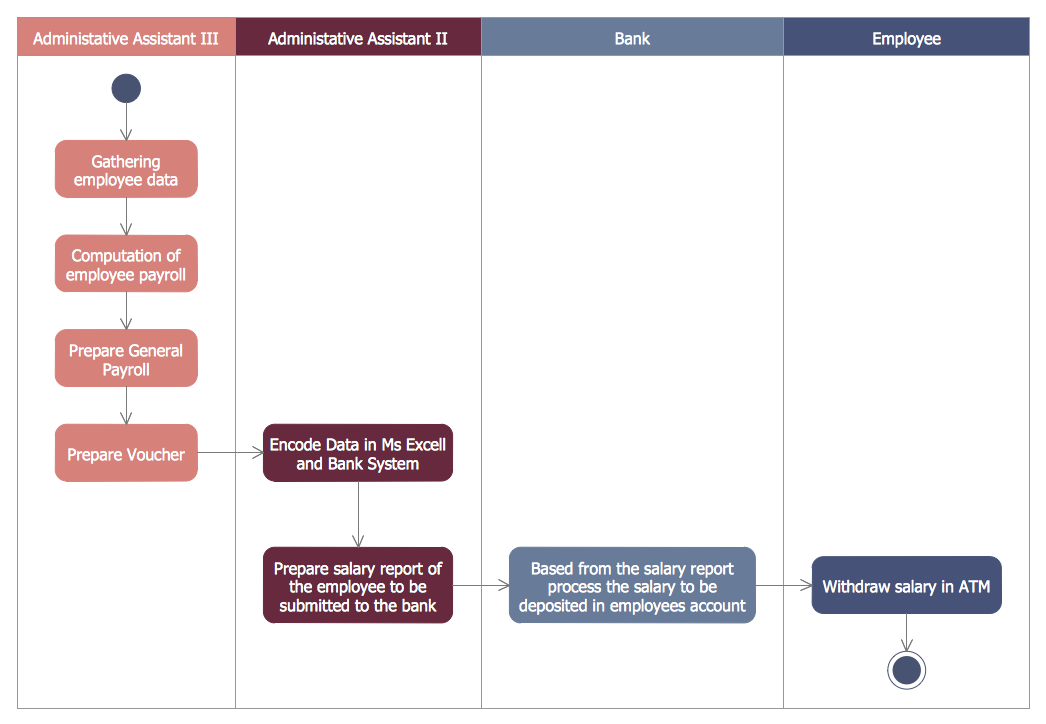## Sample 3: Bank ATM Use Case Diagram

ATM UML Diagrams sample: Bank ATM use case diagram.

This example is created using ConceptDraw DIAGRAM diagramming software enhanced with ATM UML Diagrams solution from ConceptDraw Solution Park.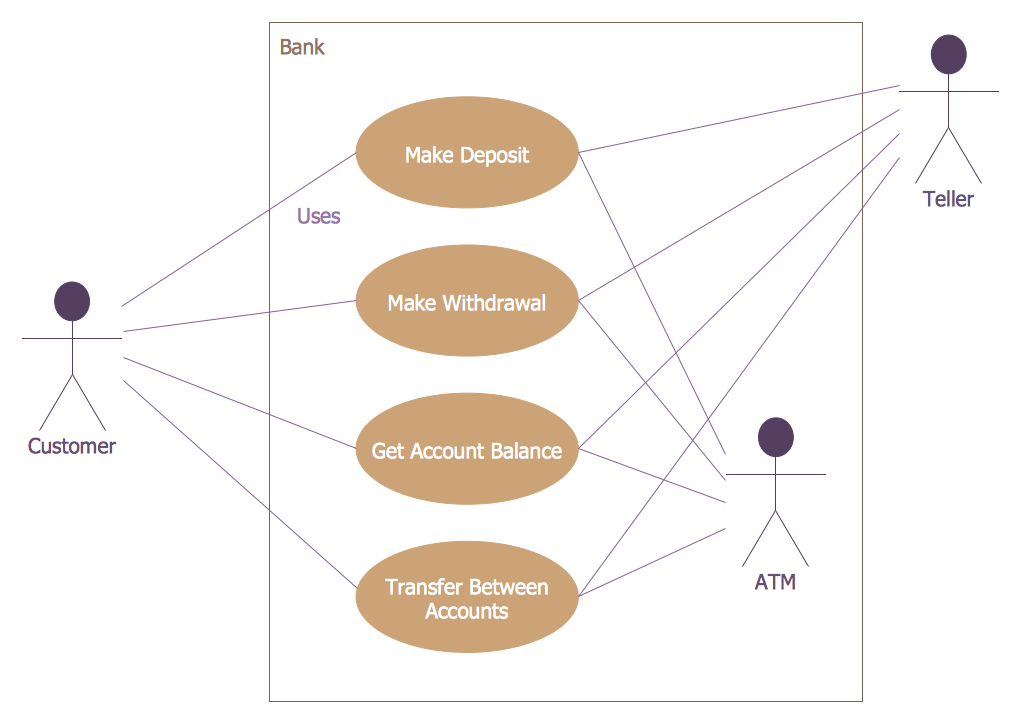## Sample 4: Class UML Diagram for Bank

ATM UML Diagrams sample: Class UML diagram for bank.

This example is created using ConceptDraw DIAGRAM diagramming software enhanced with ATM UML Diagrams solution from ConceptDraw Solution Park.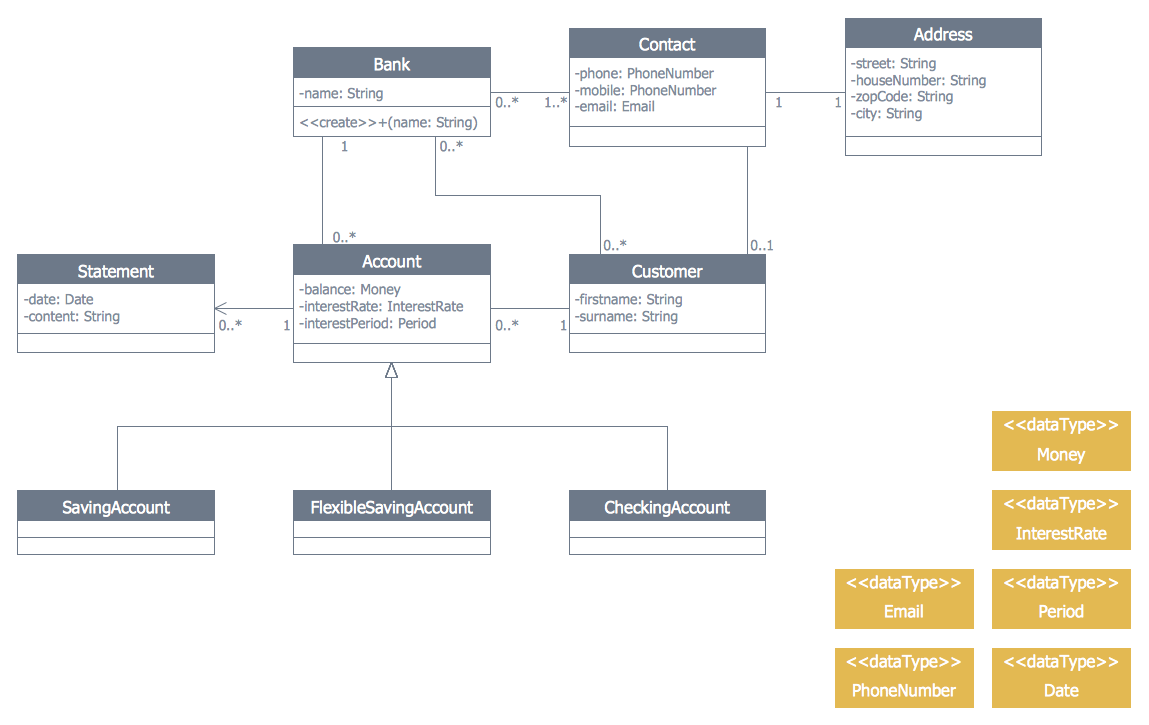## Sample 5: OPC Runtime Refinement View

ATM UML Diagrams sample: OPC runtime refinement view.

This example is created using ConceptDraw DIAGRAM diagramming software enhanced with ATM UML Diagrams solution from ConceptDraw Solution Park.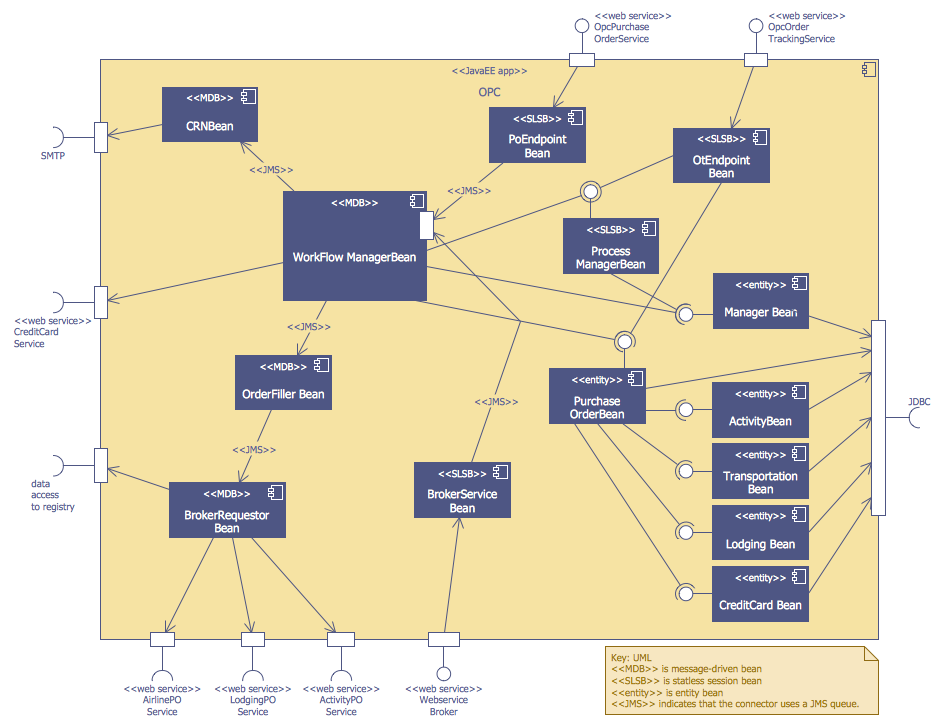## Sample 6: Purchase Order Activity Diagram

ATM UML Diagrams sample: Purchase order activity diagram.

This example is created using ConceptDraw DIAGRAM diagramming software enhanced with ATM UML Diagrams solution from ConceptDraw Solution Park.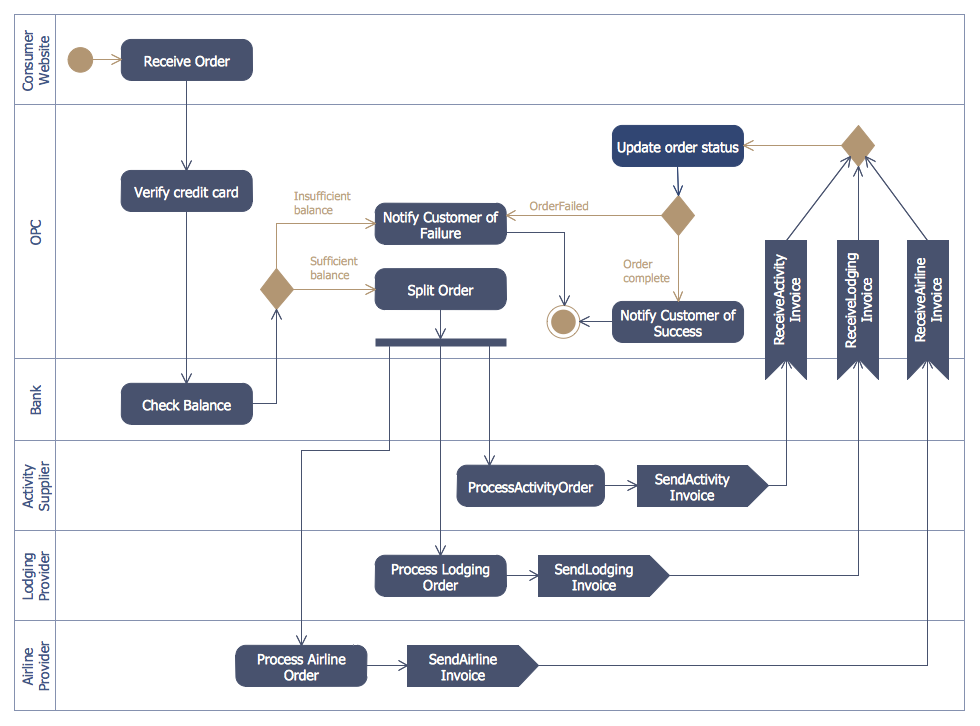## Sample 7: UML Class Diagram Bank Account

ATM UML Diagrams sample: UML class diagram bank account.

This example is created using ConceptDraw DIAGRAM diagramming software enhanced with ATM UML Diagrams solution from ConceptDraw Solution Park.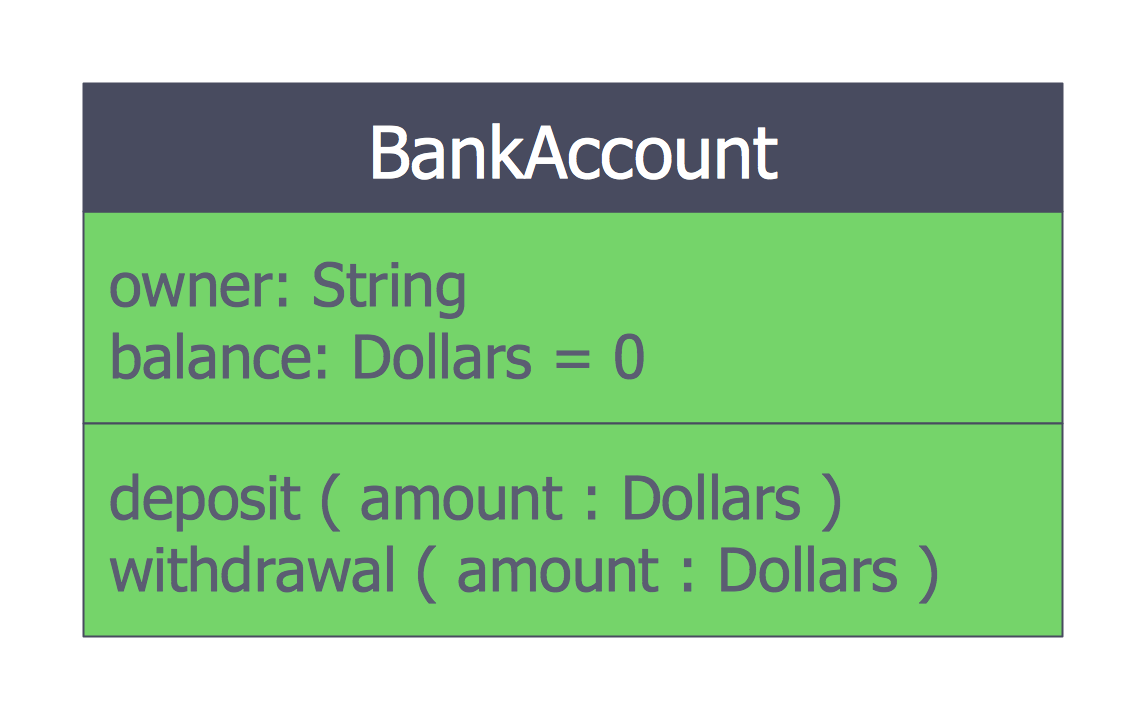## Sample 8: UML Composite Structure Diagram

ATM UML Diagrams sample: UML composite structure diagram.

This example is created using ConceptDraw DIAGRAM diagramming software enhanced with ATM UML Diagrams solution from ConceptDraw Solution Park.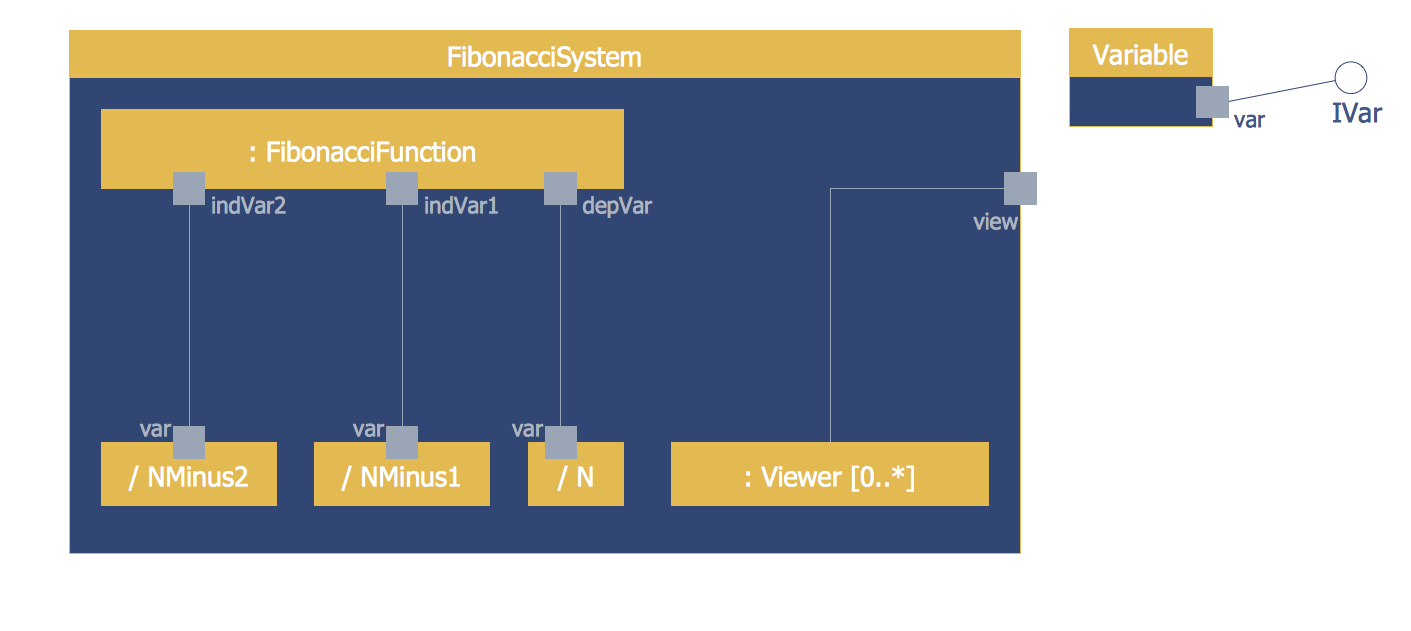## Sample 9: UML Deployment Diagram

ATM UML Diagrams sample: UML deployment diagram.

This example is created using ConceptDraw DIAGRAM diagramming software enhanced with ATM UML Diagrams solution from ConceptDraw Solution Park.## Sample 10: UML Package Diagram for Bank

ATM UML Diagrams sample: UML package diagram for bank.

This example is created using ConceptDraw DIAGRAM diagramming software enhanced with ATM UML Diagrams solution from ConceptDraw Solution Park.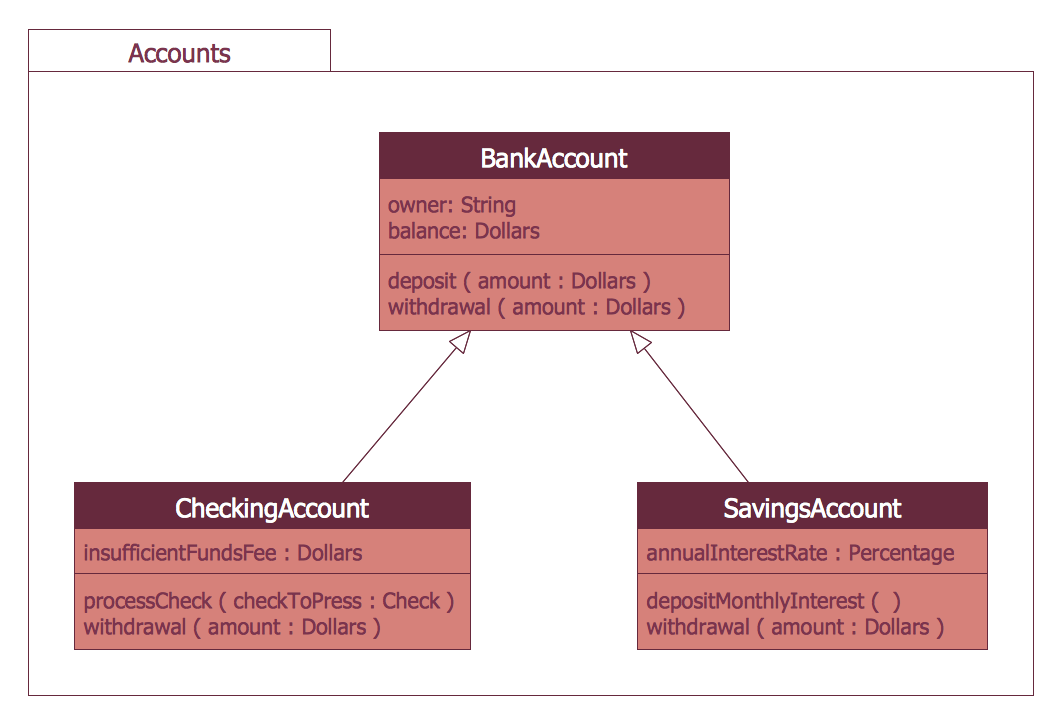## Sample 11: UML Timing Diagram

ATM UML Diagrams sample: UML timing diagram.

This example is created using ConceptDraw DIAGRAM diagramming software enhanced with ATM UML Diagrams solution from ConceptDraw Solution Park.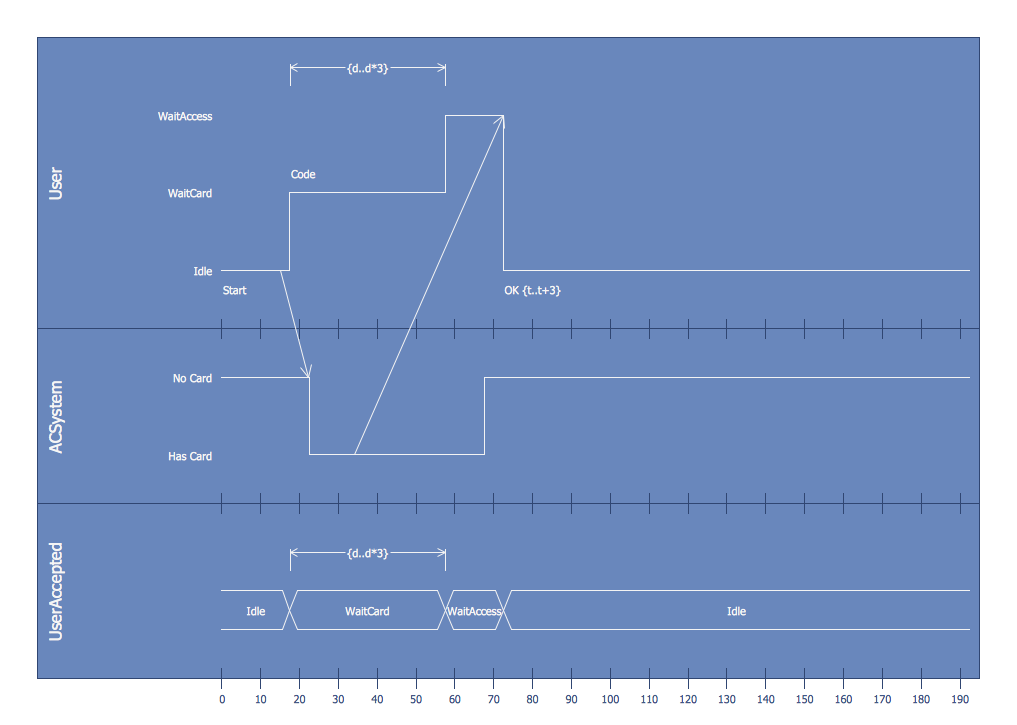All samples are copyrighted CS Odessa's.

Usage of them is covered by Creative Commons “Attribution Non-Commercial No Derivatives” License.

The text you can find at: https://creativecommons.org/licenses/by-nc-nd/3.0